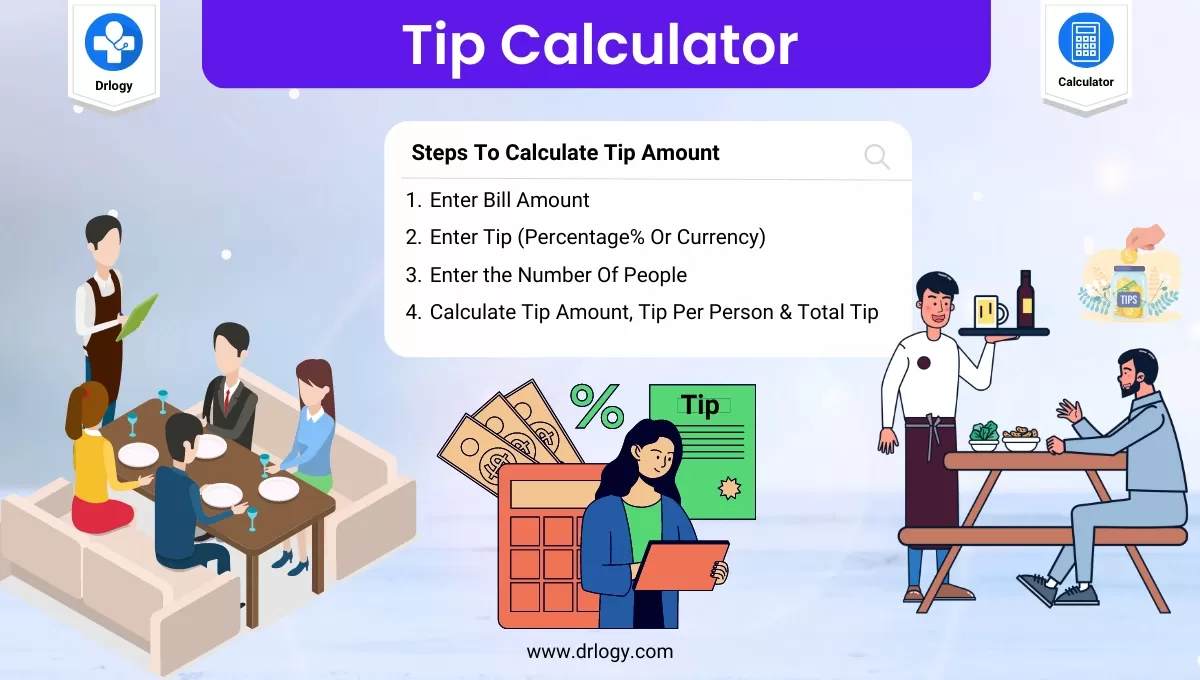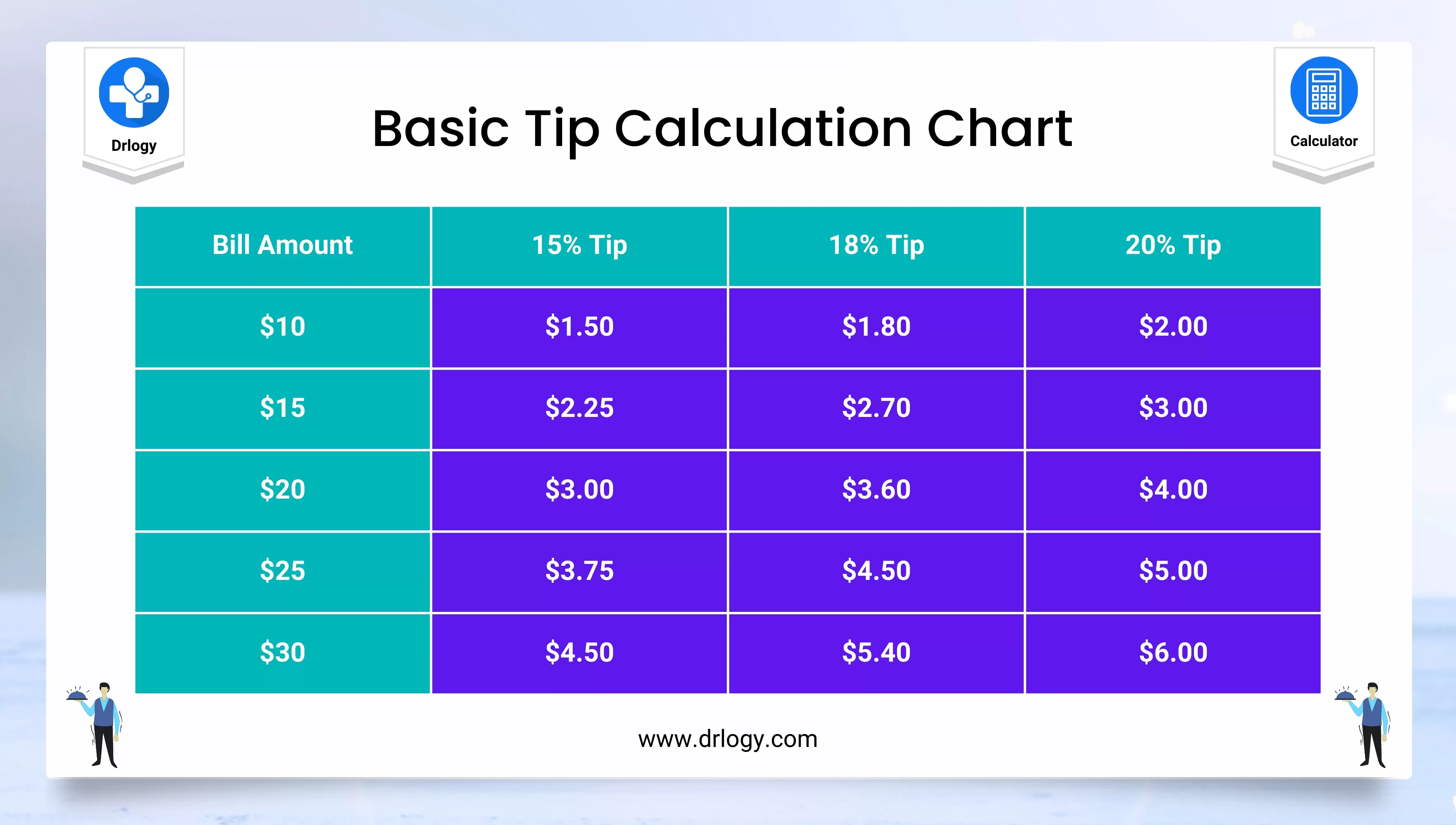• Health
• Pet
• Unit Conversion
• Medical# Tip Calculator Guide For Accurate Restaurant Tip Calculation

%

## Tip Calculator

• A tip calculator is a tool that helps you calculate the appropriate amount of tip to add to a bill at a restaurant or other service establishment.
• The amount of the tip is typically a percentage of the total bill and is left as an additional payment for the service received.
• Using a tip calculator can help you quickly and accurately determine the amount of tip to leave, based on the total bill amount and the percentage you wish to tip.
• Many tip calculators also allow you to split the total bill and tip among multiple people, which can be helpful when dining with a group.
• In addition to simplifying the math involved in calculating a tip, using a tip calculator can also help ensure that you leave a fair and appropriate amount of gratuity for the service you received.

## Steps For Tip Calculator

Here are basic guides or steps to use the tip calculator.

Enter Bill Amount

Here's an example of how to use the formula:

Let's say you have a total bill of \$50 and you want to leave a 20% tip. To calculate the tip amount, you would use the formula as follows:

Tip Amount = \$50 x 0.20 Tip Amount = \$10

So in this case, the tip amount would be \$10.

Note that the tip percentage can be customized based on your preferences and the quality of the service you received. The standard tip percentage in the United States is typically between 15% and 20%, although you may choose to tip more or less based on your experience.

## How to calculate a tip?

To calculate a tip, follow these steps:

1. Determine the total bill amount, which includes the cost of the meal or service, any applicable taxes, and any additional charges or fees.
2. Decide on the percentage of the total bill that you would like to tip. The standard tip percentage in the United States is typically between 15% and 20%, although you may choose to tip more or less based on the quality of the service you received.
3. Multiply the total bill amount by the tip percentage in decimal form. For example, if your total bill is \$50 and you want to leave a 20% tip, you would multiply \$50 by 0.20 (which is the decimal equivalent of 20%) like this:

Tip = \$50 x 0.20 Tip = \$10

So in this example, the tip amount would be \$10.

1. If you are splitting the bill and tip among multiple people, divide the total tip amount by the number of people sharing the cost to determine each person's portion of the tip.

It's important to remember that tipping is a common practice in many service industries, such as restaurants, hair salons, and taxi services, among others. Tipping helps to show appreciation for good service and is typically based on the quality of the service received.## Tip Calculator Table

Here's a tip calculator table showing different percentages for different bill amounts:

Bill Amount 15% Tip 18% Tip 20% Tip
\$10 \$1.50 \$1.80 \$2.00
\$15 \$2.25 \$2.70 \$3.00
\$20 \$3.00 \$3.60 \$4.00
\$25 \$3.75 \$4.50 \$5.00
\$30 \$4.50 \$5.40 \$6.00
\$35 \$5.25 \$6.30 \$7.00
\$40 \$6.00 \$7.20 \$8.00
\$45 \$6.75 \$8.10 \$9.00
\$50 \$7.50 \$9.00 \$10.00
\$55 \$8.25 \$9.90 \$11.00
\$60 \$9.00 \$10.80 \$12.00

15% \$100 \$15 \$115 Taxi 12% \$100 \$12 \$112 Nail Service 10% \$100 \$10 \$110 Food Delivery 15% \$100 \$15 \$115

## Tip Calculator Benefits

There are several benefits to using a tip calculator, including:

1. Accuracy: A tip calculator helps ensure that you are accurately calculating the tip amount based on the bill total and the percentage you want to tip. This can help you avoid under-tipping or over-tipping, which can be embarrassing or awkward.
2. Efficiency: A tip calculator can save time and mental energy, especially if you're splitting the bill with others or need to calculate multiple tip amounts. Instead of manually calculating each tip amount, you can simply enter the bill total and desired tip percentage into the calculator and get an instant result.
3. Fairness: A tip calculator can help ensure that each person pays a fair share of the tip when splitting the bill. By calculating each person's individual tip amount based on their portion of the bill, a tip calculator can help prevent one person from unfairly paying more or less than others.
4. Consistency: A tip calculator can help you be consistent with your tipping habits, ensuring that you're tipping the same percentage regardless of the bill total. This can help you establish good tipping habits and avoid being perceived as cheap or generous based on the bill amount.
5. Avoidance of math errors: Using a tip calculator can help prevent math errors that can occur when calculating tips manually, especially if you're in a hurry or dealing with a complicated bill. A tip calculator can provide an accurate result every time, reducing the risk of errors and potential disputes.

Summary

Overall, a tip calculator is a tool that helps users quickly calculate the amount of tip they should leave based on the total bill amount and the desired tip percentage. Check more financial calculators like this to solve your daily problems on Drlogy Calculator to get exact solution.

Reference

• How to Calculate a Tip - Video & Lesson Transcript .
• Tipping - Wikipedia .

favorite_border 2570 Likes

## Tip Calculator FAQ

### How do you do a 10% tip?

To calculate a 10% tip, you can follow these steps:

1. Determine the bill amount: Look at your restaurant bill and find the total amount due.

2. Calculate the tip amount: Multiply the bill amount by 0.10 (which is the decimal equivalent of 10%). For example, if the bill amount is \$50, you would calculate 0.10 x \$50 = \$5.

3. Add the tip amount to the bill amount: Add the tip amount you calculated in step 2 to the original bill amount to find the total amount due, which includes the tip. For example, if your bill was \$50 and you left a \$5 tip, the total amount due would be \$55.

So, if you want to leave a 10% tip, you simply multiply the bill amount by 0.10 to get the tip amount and add it to the original bill amount to get the total amount due.

### How do you calculate tips fast?

To calculate tips fast, you can use mental math and rounding techniques. Here are a few tips:

1. Round the bill amount: Round the bill amount to the nearest whole number, which will make it easier to calculate the tip mentally. For example, if the bill amount is \$47.50, round it up to \$48.

2. Use a standard percentage: Use a standard percentage, such as 15%, 18%, or 20%, depending on the quality of service you received and your personal preference.

3. Calculate the tip amount: To calculate the tip amount, simply multiply the rounded bill amount by the standard percentage. For example, if the bill amount is \$48 and you want to leave a 20% tip, multiply \$48 by 0.20, which equals \$9.60.

4. Add the tip amount to the bill amount: To find the total amount due, add the tip amount you calculated in step 3 to the original bill amount. For example, if the bill amount is \$48 and the tip amount is \$9.60, the total amount due is \$57.60.

By rounding the bill amount and using a standard percentage, you can quickly calculate the tip amount and total amount due in your head. With practice, you can become even faster at mental math and calculate tips almost instantly.

### Is 20% a big tip?

A 20% tip is considered a generous and above-average tip in most situations.

• It is commonly used as a benchmark for good service and is generally expected in upscale restaurants, where the service and quality of food are exceptional.
• However, it's important to keep in mind that the size of the tip can vary based on several factors, such as the type of service received, the level of satisfaction with the service, and personal preferences.
• Some people may choose to leave a smaller or larger tip based on these factors, and it's ultimately up to the individual to decide how much to tip.

View More Health

## Medical Calculator

View More Medical

View More Pet

## Unit Conversion Calculator

View More Unit Conversion

## Financial Calculator

View More Financial
• Home >
• Calculator >
• Tip Calculator Guide For Accurate Restaurant Tip Calculation
Drlogy®
The Power To Health